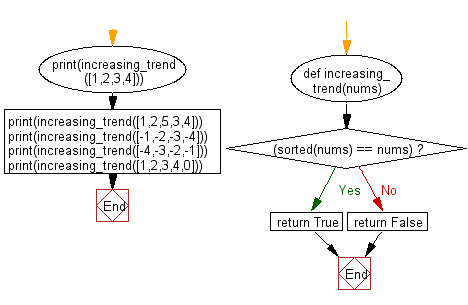﻿ Python: Check whether a sequence of numbers has an increasing trend or not - w3resource# Python: Check whether a sequence of numbers has an increasing trend or not

## Python Basic - 1: Exercise-98 with Solution

Write a Python program to check whether a sequence of numbers has an increasing trend or not.

Sample Solution:

Python Code:

``````def increasing_trend(nums):
if (sorted(nums) == nums):
return True
else:
return False

print(increasing_trend([1,2,3,4]))
print(increasing_trend([1,2,5,3,4]))
print(increasing_trend([-1,-2,-3,-4]))
print(increasing_trend([-4,-3,-2,-1]))
print(increasing_trend([1,2,3,4,0]))
``````

Sample Output:

```True
False
False
True
False
```

Pictorial Presentation:Flowchart:Python Code Editor:

Have another way to solve this solution? Contribute your code (and comments) through Disqus.

What is the difficulty level of this exercise?

Test your Programming skills with w3resource's quiz.

﻿

## Python: Tips of the Day

What is the difference between Python's list methods append and extend?

append: Appends object at the end.

```x = [1, 2, 3]
x.append([4, 5])
print (x)
```

Output:

```[1, 2, 3, [4, 5]]
```

extend: Extends list by appending elements from the iterable.

```x = [1, 2, 3]
x.extend([4, 5])
print (x)
```

Output:

```[1, 2, 3, 4, 5]
```

Ref: https://bit.ly/2AZ6ZFq